Courses

# Linear Algebra MCQ - 1

## 20 Questions MCQ Test GATE Computer Science Engineering(CSE) 2022 Mock Test Series | Linear Algebra MCQ - 1

Description
This mock test of Linear Algebra MCQ - 1 for Computer Science Engineering (CSE) helps you for every Computer Science Engineering (CSE) entrance exam. This contains 20 Multiple Choice Questions for Computer Science Engineering (CSE) Linear Algebra MCQ - 1 (mcq) to study with solutions a complete question bank. The solved questions answers in this Linear Algebra MCQ - 1 quiz give you a good mix of easy questions and tough questions. Computer Science Engineering (CSE) students definitely take this Linear Algebra MCQ - 1 exercise for a better result in the exam. You can find other Linear Algebra MCQ - 1 extra questions, long questions & short questions for Computer Science Engineering (CSE) on EduRev as well by searching above.
QUESTION: 1

### Let A be an mxn matrix and B an n*m matrix. It is given that determinant (Im + AB) = determinant (In + BA) , where Ik is the k*k identity matrix. Using the above property, the determinant of the matrix given below is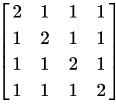Solution: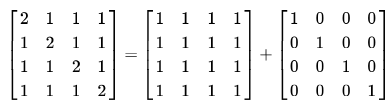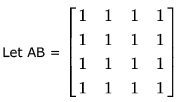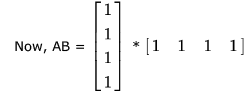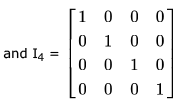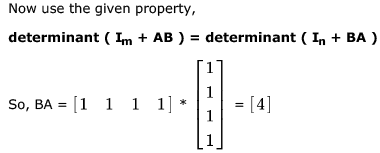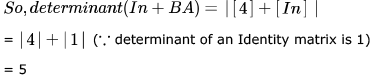QUESTION: 2

### The determinant of the matrix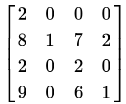Solution: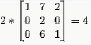QUESTION: 3

### Which one of the following does NOT equal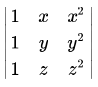?

Solution: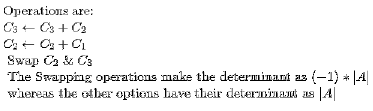QUESTION: 4

The determinant of the matrix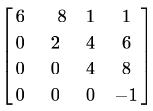Solution:

As it's upper traingular matrix ... So determinant will be product of main diagonal element.
det(A) = 6*2*4* -1 = -48.
Similar concept can be appliead , if Matrix is lower triangular or Diagonal Matrix

QUESTION: 5

The matrix of coefficients either have no solution or have infinite solutions is system of equations are

Solution:
*Answer can only contain numeric values
QUESTION: 6

If the matrix A is such that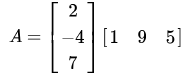then the determinant of  is equal to ______.

Solution:

Hi,
For this kind of matrices Determinant is zero.
A will be a 3x3 matrix where the first row will be 2 [1 9 5], second row will be -4 [1 9 5] and third will be 7 [1 9 5]. That is, all the rows of A are linearly dependent which means A is singular.
When matrix is singular |A| = 0

*Answer can only contain numeric values
QUESTION: 7

Two eigenvalues of a 3 x 3 real matrix P are (2 + √-1) and 3 . The determinant of P is _______

Solution:

The determinant of a real matrix can never be imaginary. So, if one eigen value is complex, the other eigen value has to be its conjugate.

So, the eigen values of the matrix will be 2+i, 2-i and 3.

Also, determinant is the product of all eigen values.
So, the required answer is (2+i)*(2-i)*(3) = (4-i2)*(3) = (5)*(3) = 15.

*Answer can only contain numeric values
QUESTION: 8

Suppose that the eigenvalues of matrix  A are 1,2, 4. The determinant of (A-1)T is _______________.

Solution: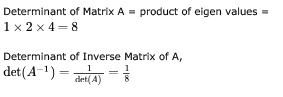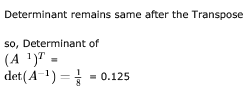QUESTION: 9

In the given matrix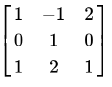, one of the eigenvalues is 1. The eigenvectors corresponding to the eigenvalue 1 are

Solution: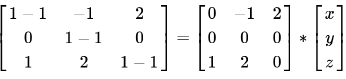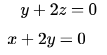now consider each of the triplets as the value of x, y, z and put in these equations the one which satisfies is the answer.
why so because an eigen vector represents a vector which passes through all the points which can solve these equations.
so we can observe that only option B is satisfying the equations.

QUESTION: 10

Consider the following 2×2 matrix A where two elements are unknown and are marked by a and b. The eigenvalues of this matrix are -1 and 7. What are the values of a and b?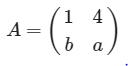Solution: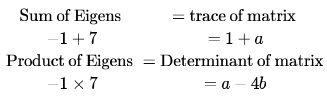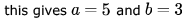*Answer can only contain numeric values
QUESTION: 11

The value of the dot product of the eigenvectors corresponding to any pair of different eigenvalues of a 4 - by - 4 symmetric positive definite matrix is ___________

Solution:

This is because eigen vectors corresponding to DIFFERENT eigen values of a REAL symmetric matrix are ORTHOGONAL to each other.
However, same eigen values they may not be.
And Dot -product of orthogonal vectors(perpendicular vectors ) is 0 (ZERO)

QUESTION: 12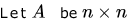real valued square symmetric matrix of rank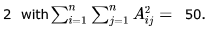Consider the following statements.

(I) One eigenvalue must be in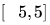(II) The eigenvalue with the largest magnitude must be strictly greater than 5

Which of the above statements about eigenvalues of  is/are necessarily CORRECT?

Solution:

Eigen values of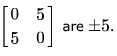Therefore second statement is false.
Since the rank of matrix  is 2, therefore atleast one eigen value would be zero for n>3.
For n= 2,  It can be proven that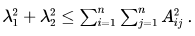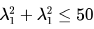Both  λ1 and λ2  would be real because  is a real symmetric matrix. Which implies that atleast one eigen value would be in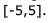Now, to prove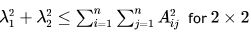matrix, let us consider the matrix is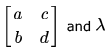is the eigen value
of this matrix.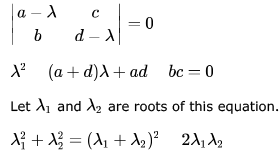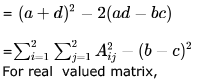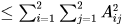(For real  symmetric matrix, b=c and < would be replaced by equal sign)

QUESTION: 13

Let A be the matrix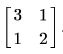What is the maximum value of xT Ax where the maximum is taken over all x that are the unit eigenvectors of A?

Solution:

x = [x1 , x2] be a unit eigen vector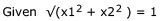i.e. x1 2 + x2 2 = 1
∴ { x is a unit Eigen vector}
x'Ax = x'Lx=  Lx'x  = L [x1,x2]' [x1,x2] = L [x12 + x22] = L(1) = L .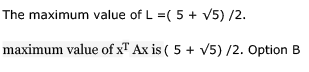QUESTION: 14

Let A be a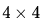matrix with eigen values -5,-2,1,4. Which of the following is an eigen value of  the matrix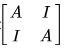, where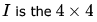identity matrix?

Solution: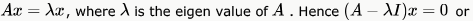So, for our given matrix, we have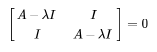This is a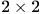block matrix where the first and last and the second and third elements are the same. So, applying the formulafor determinant of a block matrix as given here (second last case)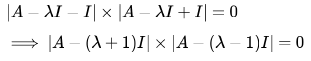Each of the eigen value of A is the solution of the equation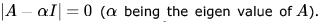. So, we can equate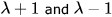to any of the eigen value of A, and that will get our value of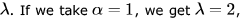and that is one of the choice. For no other choice, this equation holds. So, (c) 2 is the answer.

*Answer can only contain numeric values
QUESTION: 15

Let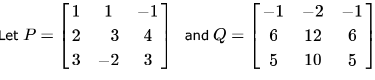be two matrices.

Then the rank of P + Q is ___________ .

Solution: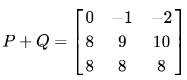det (P + Q), So Rank cannot be 3, but there exists a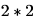submatrix such that determinant of submatrix is not 0.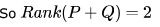QUESTION: 16

Consider the matrix as given below.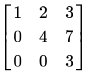Which one of the following options provides the CORRECT values of the eigenvalues of the matrix?

Solution:

The given matrix is a upper triangular matrix and the eigenvalues of upper or lower traingular matrix are the diagonal values itself. (Property)

QUESTION: 17

Which one of the following statements is TRUE about every  matrix with only real eigenvalues?

Solution:

Trace is the sum of all diagonal elements of a square matrix.
Determinant of a matrix = Product of eigen values.
A) Is the right answer. To have the determinant negative ,atleast one eigen value has to be negative(but reverse may not be true). {you can take simple example with upper or lower triangular matrices. In the case option (b) , (c) and (d) reverse is always true .}

*Answer can only contain numeric values
QUESTION: 18

The product of the non-zero eigenvalues of the matrix is ____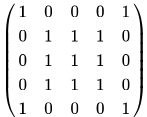Solution:

We can see that the rank of the given matrix is 2 (since 3 rows are same, and other 2 rows are also same). Sum of eigen values = sum of diagonals. So, we have two eigen values which sum to 5. This information can be used to get answer in between the following solution. Let Eigen value be X. Now, equating the determinant of the following to 0 gives us the values for X. To find X in the following matrix, we can equate the determinant to 0. For finding the determinant we can use row and column additions and make the matrix a triangular one. Then determinant will just be the product of the diagonals which should equate to 0.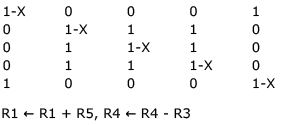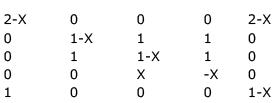Taking X out from R4, 2-X from R1, (so, X = 2 is one eigen value)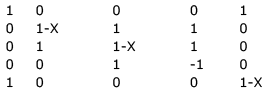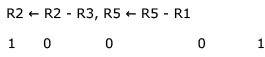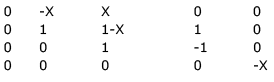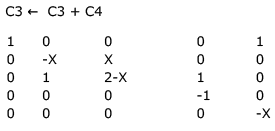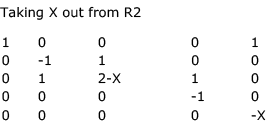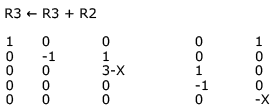Now, we got a triangular matrix and determinant of a triangular matrix is product of the diagonal.
So (3-X) (-X) = 0 => X = 3 or X = 0. So, X = 3 is another eigen value and product of non-zero eigen values = 2 * 3 = 6.

*Answer can only contain numeric values
QUESTION: 19

If the characteristic polynomial of a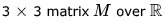(the set of real numbers) is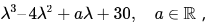and one eigenvalue of M is 2, then the largest among the absolute values of the eigenvalues of M is _______

Solution:

Given that λ = 2 is an eigen value. So, it must satisfy characterstic equation.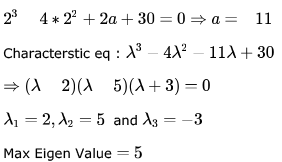*Answer can only contain numeric values
QUESTION: 20

The larger of the two eigenvalues of the matrix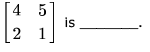Solution:

For finding the Eigen Values of a Matrix we need to build the Characteristic equation which is of the form,

A - λI
Where A is the given Matrix.
λ is a constant

I is the identity matrix.
We'll have a Linear equation after solving A - λI. Which will give us 2 roots for λ.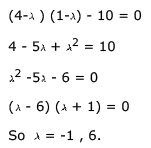6 is larger and hence is the Answer.